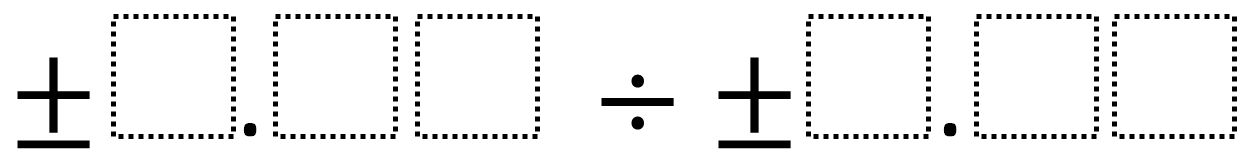Home > Grade 6 > Dividing Decimals (Middle School)

# Dividing Decimals (Middle School)

Directions: Using the digits 1 to 9 at most one time each, fill in the boxes to make the smallest (or largest) quotient.### Hint

What number does each box represent?

### Answer

No answers yet.  If you have one, post it in the comments.

Note: This problem’s difficulty can be adjusted by altering the number of digits (boxes), picking smallest or largest, or by picking either a positive, negative, or both.

Source: Robert Kaplinsky

## Subtracting Multi-Decimals

Directions: Use the digits 1 to 9, at most one time each, to fill in …

### 4 comments

1.Might it be useful to model this graphically with desmos or perhaps wolfram alpha? I’ll work on something this week.

2.Kirsten Silverman

Should probably read “Quotient” instead of sum?

•Yep. Good catch. Too much copy and pasting.

3.I feel that making a small change to find the quotient closest to 1 would add so much more opportunities for students to explore, discuss, and debate. Currently, most will try the smallest possible number (1.23) and divide it by the largest possible number (9.87) and in this case it would work, and switching the order of those 2 numbers would satisfy the prompt of finding the largest quotient. However, if they were trying to find the quotient closest to 1 there are many more combinations to try and better conversations will surface. What does it mean when the quotient is 1, and how can I get as close to that as possible with these constraints? Does the order matter?